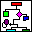#Data-Stream-Network

## Goals

There are no standard ways for analyzing data, and in particular timeseries. Known analysis methods have very often to be specially adapted for solving a given problem. Since in general timeseries represent only a time-directional projection of complex dynamic systems, their analysis requires experience and creativity. By visualizing appropriately the data, experts are able to recognize crucial information only by merely watching the presentation. Many algorithms need to be tested with different parameter-settings. Since several algorithms are to be connected in series, the parameter space to be searched can easily overgrow the attention span of the user.
The main goal followed when developing this program was to give the user the possibility for handling these problems in a quick, simple, and sensitive way. Our approach is somewhat similar to the strategy followed by other public domain and/or professional tools like Khoros or Irix Explorer but reduced to what we think is a necessary minimum. For instance, we provide the possibility of graphically (and functionally) connecting algorithmic blocks but we restrict here to tree-like structures (no loops are allowed). The data and the algorithms are represented by icons which can be graphically connected on a work-canvas using the mouse and a predefined list of icons. After building big data-streams (screen-shot) with this method, the calculation is started by clicking on the desired (usually the last connected) output data. If later some parameters or data are changed up-stream, a new click on the desired output will automatically update all necessary data. Each data type has an automatically graphic display format, which in this variant calls the public domain program Gnuplot.

## Integrated Algorithms

• Generating some Standard Test-Timeseries
• Normal and uniform Noise,
• The Logistic and Hénon Map, the Lorenz Model, the Standard Map,
• ARMA
• Step,- Sinus-Cosine, polynomial Functions
• Methematical Pendulum
• Binary Timeseries Analysis
• Ten different Algorithms
• Elementary Manipulations of one or more Timeseries
• Split, Append, Time-Shift, Scalar Operator, Logarithms, Rounding
• Espectation Value, Variance, Data Scaling
• Addition, Subtraction, Multiplication, and Division of two Timeseries
• Advanced Manipulation and Analysis of a Timeseries
• Moving Averages with Different Filters
• Interpolation
• Difference- and other Filters
• Correlations
• Linear and Polynominal Extrapolation
• Statistics
• Distributions, Histogram
• Multivariate Analysis of Timeseries
• Time-Delay Method
• Cross-Correlation Matrix with Block Sorting
• Principal Component Analysis and affine Transformations
• Frequency Domain Aanalysis
• Fast Fourier Transformation (^-1)
• Continuous Fourier Transformation (^-1)
• Bandfilters
• Maximum Entropy Spectrum
• Main Frequency Analysis
• Schreiber-Filter
• Discrete Wavelet Transformation (^-1)
• Prediction
• ARMA
• Nearest Neighbour Prediction
• Clustering in Phase Space
• Estimation of Lyaponov-exponents with RFB
• Autoregressive Prediction
• Graphic Output (2D and 3D) (exportable to documents via clipboard )
• Matrices
• Principal-Components
• Timeseries
• Fourierspectrum
• Waveletspectrum
• Cluster
• Histogram
• Sound (exporting a timeseries in wave-file format)

## Availability

• Hardware:
• Any IBM-PC compatible with Proc.>= 80386, RAM>=8 MB, Harddisk>=5 MB, color-display
• Software